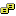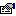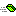## SymmetricAlgorithm

Provides a standard interface for utilizing symmetric algorithms.

### Public:

#### Types:

NameDescriptionCipherMode A defined set of cipher modes the algorithms can use.PaddingMode A defined set of padding modes used to fill the end of non-blocksize length data blocks. For example if an algorithm has a blocksize of 8 bytes and the data is 3 bytes in length, then 5 bytes of padding must be applied.

#### Properties:

NameDescriptionBlockSize (get) Gets the block size, in bits, used by the cryptographic operation.BlockSize (let) Sets the block size, in bits, used by the cryptographic operation.FeedbackSize (get) Gets the feedback size, in bits, used by the cryptographic operation.FeedbackSize (let) Sets the feedback size, in bits, used by the cryptographic operation.IV (get) Gets the initialization vector (IV) for the symmetric algorithm.IV (let) Sets the initialization vector (IV) for the symmetric algorithm.Key (get) Gets the secret key for the symmetric algorithm.Key (let) Gets the secret key for the symmetric algorithm.KeySize (get) Gets the size of the key, in bits, used by the symmetric algorithm.KeySize (let) Gets the size of the key, in bits, used by the symmetric algorithm.LegalBlockSizes (get) Gets the block sizes, in bits, that are supported by the symmetric algorithm.LegalKeySizes (get) Gets the key sizes, in bits, that are supported by the symmetric algorithm.Mode (get) Gets the mode for operation of the symmetric algorithm.Mode (let) Sets the mode for operation of the symmetric algorithm.Padding (get) Gets the padding mode used in the symmetric algorithm.Padding (let) Gets the padding mode used in the symmetric algorithm.

#### Methods:

NameDescriptionClear Releases all resources used by the SymmetricAlgorithm class.CreateDecryptor When implemented by a class, creates a symmetric decryptor object with the specified Key property and initialization vector (IV).CreateEncryptor When implemented by a class, creates a symmetric encryptor object with the specified Key property and initialization vector (IV).Equals Returns if this instance and the value are equal.GenerateIV When implemented by a class, generates a random initialization vector (IV) to use for the algorithm.GenerateKey When implemented ba a class, generates a random key (Key) to use for the algorithm.GetHashCode Returns a semi-unique value that represents this instance.ToString Returns a string representation of this instance.ValidKeySize Determines whether the specified key size is valid for the current algorithm.

#### Remarks

A symmetric algorithm is one that uses the same Key and Initialization Vector to both encrypt and decrypt data.

For one of the symmetric algorithms to work, a Key and Initialization Vector needs to be supplied to the Crypto Service Provider.

RijndaelManaged, DESCryptoServiceProvider, RC2CryptoServiceProvider, and TripleDESCryptoServiceProvider are implementations of symmetric algorithms.